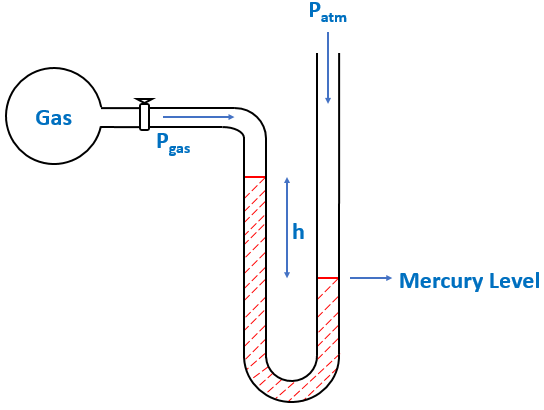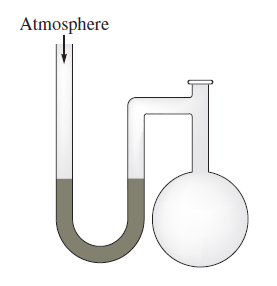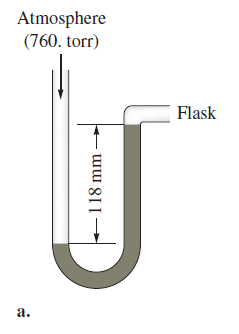# Problem: A diagram for an open-tube manometer is shown below.If the flask is open to the atmosphere, the mercury levels are equal. For each of the following situations where a gas is contained in the flask, calculate the pressure in the flask in torr, atmospheres, and pascals.a. If the open-tube manometer in contains a nonvolatile silicone oil (density = 1.30 g/cm3) instead of mercury (density = 13.6 g/cm3), what are the pressures in the flask in torr, atmospheres, and pascals?

###### FREE Expert Solution

We’re being asked to determine the pressures in the flask in torr, atmospheres, and pascals, if the open-tube manometer in contains a nonvolatile silicone oil.

A manometer allows you to determine the pressure of a gas (Pgas) sample by using the atmospheric pressure (Patm) and the difference in the height of mercury (h).

When Patm is pushing harder than  Pgas$\overline{){{\mathbf{P}}}_{\mathbf{g}\mathbf{a}\mathbf{s}}{\mathbf{=}}{{\mathbf{P}}}_{\mathbf{a}\mathbf{t}\mathbf{m}}{\mathbf{-}}{\mathbf{h}}}$

From the illustration given, the atmospheric pressure (Patm) pushing the silicone mercury harder than the pressure of the gas (Pgas) giving a height difference of 118 mmHg.

87% (263 ratings)###### Problem Details

A diagram for an open-tube manometer is shown below.If the flask is open to the atmosphere, the mercury levels are equal. For each of the following situations where a gas is contained in the flask, calculate the pressure in the flask in torr, atmospheres, and pascals.a. If the open-tube manometer in contains a nonvolatile silicone oil (density = 1.30 g/cm3) instead of mercury (density = 13.6 g/cm3), what are the pressures in the flask in torr, atmospheres, and pascals?

Frequently Asked Questions

What scientific concept do you need to know in order to solve this problem?

Our tutors have indicated that to solve this problem you will need to apply the Manometer concept. If you need more Manometer practice, you can also practice Manometer practice problems.

What textbook is this problem found in?

Our data indicates that this problem or a close variation was asked in Chemistry: An Atoms First Approach - Zumdahl Atoms 1st 2nd Edition. You can also practice Chemistry: An Atoms First Approach - Zumdahl Atoms 1st 2nd Edition practice problems.